##Projectivity

This is defined as an invertible transformation of the projective plane onto itself, that preserves lines. In the standard representation of the projective plane as the set of lines [x1, x2, x3] of R3, a projectivity is a class of invertible matrices [A], consisting of the non-zero multiples of a non singular 3x3 real matrix A. With such a representation, point x = [x1, x2, x3] is transformed to [A][x] = [Ax]. Projectivities (some times I call them also homographies) are defined by giving four points {Pi} and their images {Qi}, both quadrupples in general position, meaning, for each quadrupple, that each subset of three points is not collinear.
Algebraically, if Pi is represented by [pi] = [p1i, p2i, p3i]t (column vector), and Qi correspondingly with [qi] = [q1i, q2i, q3i]t, then the corresponding A has the form A = QKP-1, K being a diagonal matrix: K = diag{k1, k2, k3} and P, Q being the matrices with columns {pi}, {qi} respectively, for i=1,2,3.
The arbitrary constants ki are determined by the fourth point and the condition:
QKP-1p4 = k4q4.
This represents a system of 3 homogeneous equations with four unknowns (unknowns are k1, ... , k4), and rank three, thus accepting a solution depending on one parameter (k1, ... , k4) = t(k01, ..., k04). This determines a unique class [A] = [QKP-1].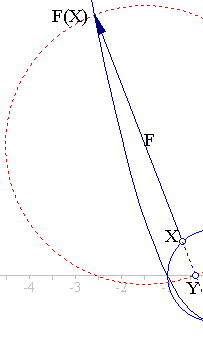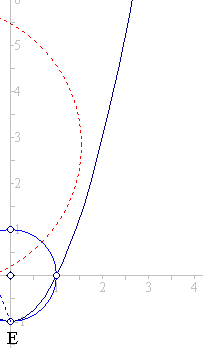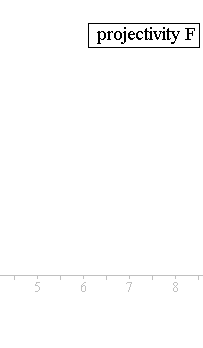As an example, construct the projectivity fixing the points [1,0,1]t, [-1,0,1]t, [0, -1,1]t and mapping p4 = [0,1,1]t to the point at infinity along (0,1) which is q4 = [0,1,0]t. The corresponding matrices are: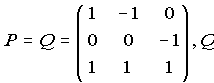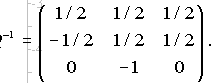Equation QKP-1p4 = k4q4, takes the form: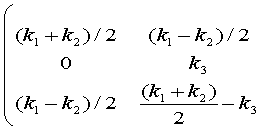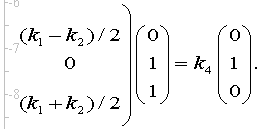And has the solutions (k1, k2, k3, k4) = t(1,1,2,2), thus completely determining A, which is: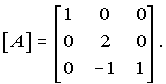The projectivity defined through that matrix maps the points (x,y) of the unit circle: x2 + y2 = 1, to: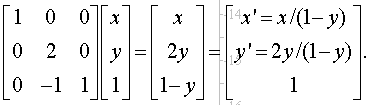(x', y') satisfying y' = x'2 - 1, which is a parabola (shown above). The easily shown vanishing of the determinant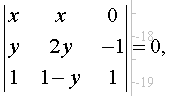proves that this projectivity F has for every point X(x,y), the image-point F(X)=(x',y') and the point E(0,-1) collinear. Further, by inspecting [A], we see that (i) the x-axis and point E are the only fixed points of F, whereas the whole line y = 1 maps to the line at infinity. A further computation of the cross-ratio [E,X,F(X),Y], where Y the intersection point of line EX with the x-axis, shows that this is -1 (harmonic). F coincides not with the perspectivity with center at E and axis the x-axis, and homology coefficient equal to 2.
In this discussion I have consistently and often used the projectification of the euclidean plane, which identifies the point (x,y) of euclidean space with the point [x,y,1] of the projective space, and inversely it identifies point [x,y,z] of the projective space with point (x/z, y/z) of the euclidean space (provided [x,y,z] is not a point on the line at infinity, meaning that z is non-zero).
The above image dictates a procedure of generation of parabolas, studied in ParabolaProjectFromCircle.html .